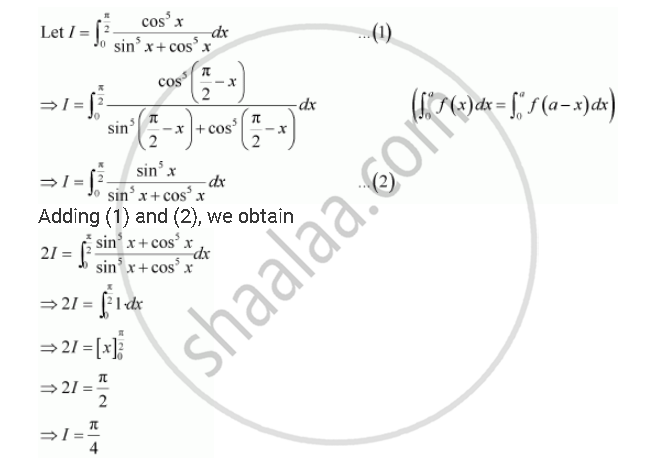Share

# By Using the Properties of Definite Integrals, Evaluate the Integrals Int_0^(Pi/2) (Cos^5 Xdx)/(Sin^5 X + Cos^5 X) - CBSE (Science) Class 12 - Mathematics

ConceptProperties of Definite Integrals

#### Question

By using the properties of definite integrals, evaluate the integrals

int_0^(pi/2)  (cos^5  xdx)/(sin^5 x + cos^5 x)

#### SolutionIs there an error in this question or solution?

#### Video TutorialsVIEW ALL 

Solution By Using the Properties of Definite Integrals, Evaluate the Integrals Int_0^(Pi/2) (Cos^5 Xdx)/(Sin^5 X + Cos^5 X) Concept: Properties of Definite Integrals.
S